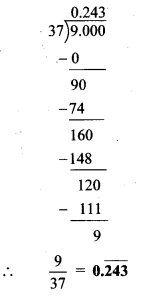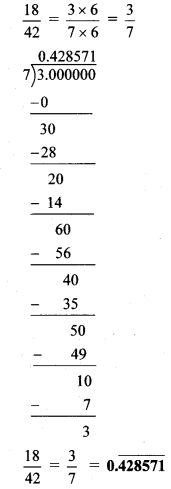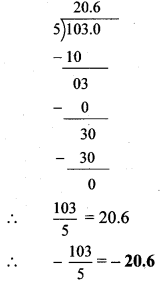# Maharashtra Board 8th Class Maths Practice Set 1.3 Solutions Chapter 1 Rational and Irrational Numbers

Balbharti Maharashtra State Board Class 8 Maths Solutions covers the Practice Set 1.3 8th Std Maths Answers Solutions Chapter 1 Rational and Irrational Numbers.

## Practice Set 1.3 8th Std Maths Answers Chapter 1 Rational and Irrational Numbers

Question 1.
Write the following rational numbers in decimal form.
i. $$\frac { 9 }{ 37 }$$
ii. $$\frac { 18 }{ 42 }$$
iii. $$\frac { 9 }{ 14 }$$
iv. $$-\frac { 103 }{ 5 }$$
v. $$-\frac { 11 }{ 13 }$$
Solution:
i. $$\frac { 9 }{ 37 }$$ii. $$\frac { 18 }{ 42 }$$iii. $$\frac { 9 }{ 14 }$$iv. $$-\frac { 103 }{ 5 }$$v. $$-\frac { 11 }{ 13 }$$